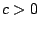# On growth rates of closed sets of permutations, set partitions, ordered graphs and other objects

Martin Klazar

Charles University, Prague

Ifis a closed set of finite permutations (i.e., a lower ideal inwhereis the set of all finite permutations andis the standard containment ordering) anddenotes the set of all-permutations in, then the counting functionis subject to various dichotomies and restrictions forbidding many functions to have this form; this was shown (Electronic J. of Combinatorics, 2003) by T. Kaiser and M. Klazar. For example, eitherfor alloris eventually constant, or--another dichotomy--eitherfor allwith a constantorfor all, whereare the Fibonacci numbers.

In my talk I will present generalizations and extensions of these results to other classes of objects (like those mentioned in the title) and other containments, and I will discuss a general approach to obtain them uniformly as instances of a general metaresult.

2005-05-23